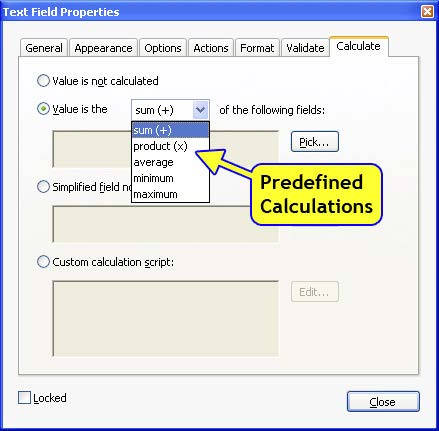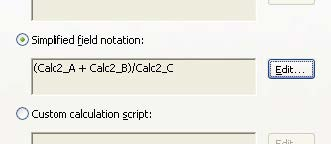# How to do (not so simple) form calculations

## Learn about the three options for creating form-field calculations using Acrobat JavaScript.

By July 2, 2006Scope: All Acrobat versions
Skill Level: Beginner
Prerequisites: Familiarity with Acrobat Professional

Acrobat provides three options for creating form-field calculations:

• Predefined calculations
• Simplified field notation
• Custom JavaScript calculations

In reality, all three are JavaScript, but for the first two, Acrobat builds the JavaScript code for us. Calculations are only available for text fields and are accessed through the Text Field Properties dialog.

To enter a calculation:

1. Activate the Select Object Tool. In Acrobat 9 and earlier, this tool is accessed on the Advanced Editing toolbar. In Acrobat X, it is available in both normal operating mode and in form edit mode. Figure 1 shows screen shots of both modes.Figure 1: Activating the Select Object Tool causes the form fields to be highlighted and the field names to be displayed.

2. Right-click the cursor on the text field where the calculation result will be displayed and select Properties from the popup menu. This action displays the Text Field Properties dialog (Figure 2).Figure 2: Text-field calculation options/Selecting a predefined calculation

3. On the Text Field Properties dialog, select the Calculate tab.
4. Select the desired calculation option: Predefined, Simplified field notation, or Custom calculation script.
5. Enter the actual calculation data. This data will, of course, be different for each of the different types of calculations. The accompanying example file, SimpleCalcExample.pdf has one example for each.

## Predefined calculations

The first option on the Calculate tab is for the predefined calculations. To use this option, simply select one of the calculations from the drop-down list, click on the Pick… button and select the fields to be used as inputs (Figure 2).

## Simplified field notation

The predefined calculations are rather limited, for example, there is no division or subtraction. The simplified field notation allows the creation of much more complex calculations. It uses a notation similar to how a calculation would ordinarily be written, i.e., using the regular math symbols, + (addition), -(subtraction), *(multiplication) and /(division). Field names are used as operands. In the example file the equation:

D = (A + B)/C

is calculated by entering (Figure 3)

(Calc2_A + Calc2_B) / Calc2_C

Where Calc2_A, Calc2_B, Calc2_C are the names of text fieldsFigure 3: Syntax for using the simplified field notation

This notation is limited to calculations that only use the standard four math operators. It also does not handle form field names that include punctuation or spaces. Special characters are, in fact, the main reason calculations using this method fail, so let me repeat the restriction again. Simplified field notation cannot operate on any field name that includes spaces or punctuation.

## Custom calculation script

To create more complex logic or mathematics the third option, Custom calculation script must be used. This option requires entering JavaScript and gives full access to all the fields (and other features) on the PDF file, as well as the rich math features in the JavaScript language. However, it also requires use of the full Acrobat JavaScript syntax which makes the calculations significantly longer. For example, the following code solves the same equation used in the simplified field notation example above.

```   event.value = ( this.getField("Calc2_A").value + this.getField("Calc2_B").value ) / this.getField("Calc2_C").value;
```

The first thing to notice is that the calculated value is assigned the variable event.value. The value of this variable is assigned to the field when the calculation is completed. All calculation scripts must assign a value to the event.value variable. The second thing to note is that the input field values are explicitly acquired. Take for example the input value

this.getField("Calc2_A").value

The first part of this code segment, this.getField("Calc2_A"), acquires the Field Object for the field named Calc2_A. The actual value for the field is obtained from the value property. Even experienced JavaScript programmers sometimes forget to append the value property, so watch out for this common omission and carefully inspect all the code.

While this is all you need to know to get started using calculations in Acrobat forms, there is more. If you want to use advanced features or do something more complex than described in the example file SimpleCalcExample.pdf , can find information in both the Acrobat JavaScript Scripting Reference1. You may also want to look up the core JavaScript Math object, which provides details on advance mathematical functions. Find information on this object in any standard JavaScript reference, or on line at the Mozilla developer site.

 Related topics: PDF Forms, JavaScript Top Searches: Convert existing forms to fillable PDFs, fill and sign any form, Edit PDF, create PDF, Action Wizard

Comments for this tutorial are now closed.

#### Thom Parker

10, 2015-11-23 23, 2015

Loretta, Yes this is possible.  The event.rc property blocks a calculation script from setting the value, allowing the value to be entered from a different source. So your calculation script could look like this

var price = this.getField(“Price”).value;
var quantity = this.getField(“Quantity”).value;
event.rc = (price == “”) && (quantity == “”);
event.value = price * quantity;

Note that the event.rc property is also used to set the readonly property, so that the user is blocked from trying to enter a value when the price and quantity values are present.

#### Loretta

3, 2015-11-21 21, 2015

Is this possible to create in Acrobat?

I would like to write a formula to calculate the product of two fields if there is a value present.  If there is not a value present then I would like to use the same field to hand enter an override value.

Example:  Mileage * mile rate =  Product.  If both mileage and mile rate are blank, then the field will be open to accept a random unrelated number.

#### Thom Parker

12, 2015-07-18 18, 2015

Abram,  When you change a value on a PDF, such as your summation, the calculation does not subtract, it recalculates. The issue then is with your calculation. The most likely problem is the calculation order. The final summation field needs to be at the end of the calculation order so that it calculates last, after everything else.

The menu item for setting the calculation order is in a different location in every version of Acrobat, you’ll need to look at the documentation for your version to find it.

#### Abram

7, 2015-07-14 14, 2015

Hi Guys

Please help i’ve created a interactive pdf which has a unit price, quantity(qty) and price(Which is the product of unit price and qty).
Now all these prices add up to a total price at the bottom which works, but the problem is it only adds up and doesn’t minus if i had changed the qty of an item to less, the total price at the problem doesn’t substract. Please help.

#### Thom Parker

5, 2014-12-15 15, 2014

Deborah Carpenter, please post a detailed explanation of your issue to the forums. This said, one possible issue with the automatic calculations (non-custom) is field naming. Field names must not include spaces or special characters. Just plain text. If this isn’t the issue then you’ll need to go to the forum.

#### Thom Parker

5, 2014-12-15 15, 2014

Kelli, the answer is Yes, sort of;)  You can include any kind of form field data in a calculation, just as long was the script knows which fields to include. The key to all programming is the deterministic nature of the problem at hand. If you can write it out as a deterministic sequence of actions, then it can be programmed.

#### Deborah Carpenter

2, 2014-11-25 25, 2014

I have used the subtraction calculation is forms before and had no problem. This new form I have created is not calculating using the same method. Please advise.

#### Kelli

9, 2013-12-06 06, 2013

Is it possible to run a custom calculate script that identifies a pre-determined amount of non-selected check boxes that counts down every time a check box is selected?

#### Patty Friesen

1, 2013-03-06 06, 2013

Hi Jane,

If you have a question regarding calculations, can you please post it in the Answers Q&A and one of our experts will respond:

Thanks, Patty

#### Jane Cheechoo

2, 2013-02-26 26, 2013

I tried the simplied (field notation)
should be Travel Advance \$42.00 minus Travel Claim \$122.00= \$80.00
this is the Edit TravelAvance-TravelClaim
\$1.00 is what I got.

#### Patty Friesen

8, 2012-12-10 10, 2012

Hi Clayton,

Can you post this question to our experts here in the JavaScript category:

so either Thom or other JavaScript experts can help you interactively and other members of the community can benefit from the question and answer?

Thanks,
Patty

#### Clayton Hauk

6, 2012-12-07 07, 2012

If my formula is this “(((Text44 - Text18)- Text49) - Text45) * .32)”
How would this translate into either simple or custom?

Comments for this tutorial are now closed.